Define work?

Difficulty: Medium

Work:

Work is done when a force acting on a body displaces it in the direction of a force. Work is a scalar quantity. It depends on the force acting on a body, displacement of the body, and the angle between them.

Work done = Force x displacement

Or   W = F S ......... (i)

$W = F_{x}\times S$
$W = \left(F\cos\theta\right)S$
$W = FS \cos\theta$ .......(ii)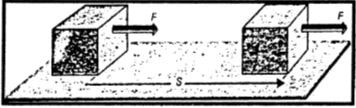Unit of work:

SI unit of work is the joule (J). it is defined as

The amount of work is one joule when a force of one newton displaces a body through one meter in the direction of the force.

Thus        $1 J = 1 N\times1m$

Derive the relation for work done by a force inclined with the displacement?

Difficulty: Medium

Let the force F is making an angle q with the surface on the body is moved.

Resolving F into its perpendicular components Fx and Fy as;

$F_{x}=F\cos\theta$

$F_{y}=F\sin\theta$

In case when force and displacement are not parallel then only the x-component $F_{x}$ parallel to the surface causes the body to move on the surface and not the y - component $F_{y}$

Hence  W =  $F_{x}\times S$

$W= \left(F\cos\theta\right)S$

$W = FS \cos\theta$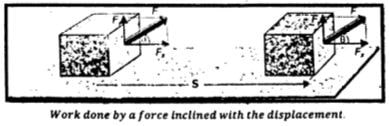Mini Exercise

A crate is moved by pulling the rope attached to it. It moves 10 m on a straight horizontal road by a force of 100 N. How much work will be done if?

1. The rope is parallel to the road

Solution:        Force = 100 N

Distance = S = 10 m

Work = W = ?

$W=F\times S$

$W = 100 x 10 = 1000 J$

2. The rope is making an angle of 300 with the road.

Solution:            Force = 100 N

Distance = S = 10 m

$\theta =30\circ$

Work = W = ?

$W=FS cos\theta$
$W = 100\times10 cos(30\circ)$
$W = 1000\times (0.866) = 866 J$

Define energy, and give two types of mechanical energy.

Difficulty: Easy

Energy:

A body possesses energy if it is capable to do work.

Types of mechanical energy:

Mechanical energy possessed by a body is of two types: kinetic energy and potential energy.

Why do we need energy?

Difficulty: Medium

Need for energy:

Energy is a fundamental concept in science. It links almost all the natural phenomena. When we say that a body has energy, we mean that it can do work, so it possesses energy. The energy from running water can be used to run the water mills or water turbines.

Energy exists in various forms such as mechanical energy, heat energy, light energy, sound energy, electrical energy, chemical energy, nuclear energy, etc. Energy can be transformed from one form into another.

Define K.E. and derive its relation.

OR

Prove that K.E. =   K.E. =  $\frac{1}{2} m v^{2}$

Difficulty: Medium

Kinetic Energy:

The energy possessed by a body due to its motion is called its kinetic energy.

Derivation of K.E:

Consider a body of mass m moving with velocity v. The body stops after moving through some distance S due to some opposing force such as the force of friction acting on it. The body possesses kinetic energy and is capable to do work against opposing force F until all of its kinetic energy is used up.

K.E. of the body = Work done by it due to motion

K.E      =        FS.......... (i)

Vi         =        v

Vf        =        0

As                                              F =  ma

A   =   $-\frac{F}{m}$

Since motion is opposed, hence, a is negative. Using 3rd equation of motion:

$2aS=V_f^2- V_i^2$

$2 (- F/m) S = (0)2 – (v)2$

F S =$\frac{1}{2} m v^{2}$........(ii)

From Eq. (i) and (ii), we get

K.E. =  $\frac{1}{2} mv^{2}$.......(iii)

Equation (iii) gives the K.E. possessed by a body of mass m moving with velocity v.

Define potential energy and derive its relation?

Difficulty: Easy

Potential Energy:

The energy possessed by a body due to its position is known as its potential energy.

Derivation of P.E:

Let a body of mass m be raised through height h from the ground. The body will acquire potential energy equal to the work done in lifting it to height h.

Thus, Potential energy  P.E. =$F\times h$
= $w\times h$

(Here weight of the body = w = mg)

P.E.= w h= mgh………(i)

Thus, the potential energy possessed by the body concerning the ground is much and is equal to the work done in lifting it to height h.

List the different forms of energy with examples?

Difficulty: Easy

Forms of Energy:

Energy exists in various forms. Some of the main forms of energy are given:

1. Mechanical energy
2. Heat energy
3. Electrical energy
4. Sound energy
5. Light energy
6. Chemical energy
7. Nuclear energyDescribe mechanical energy with examples?

Difficulty: Easy

Mechanical energy:

The energy possessed by a body both due to its motion or position is called mechanical energy.

Examples:

Water running down a stream, wind, a moving car, a lifted hammer, a stretched bow, a catapult or a compressed spring, etc. possesses mechanical energy.

Describe heat energy with examples?

Difficulty: Easy

Heat energy:

Heat is a form of energy given out by hot bodies. A large amount of heat is obtained by burning fuel. Heat is also produced when motion is opposed by frictional forces. The foods we take provide us with heat energy.

Examples:

The Sun is the main source of heat energy.

Describe electrical energy with examples?

Difficulty: Easy

Electrical energy:

Electricity is one of the widely used forms of energy. Electrical energy can be supplied easily to any desired place through wires.

Examples:

We get electrical energy from batteries and electric generators. These electric generators are run by hydropower, thermal or nuclear power.

Describe sound energy with examples?

Difficulty: Easy

When you knock at the door, you produce sound. A sound is a form of energy.

Examples

It is produced when a body vibrates; such as the vibrating diaphragm of a drum, vibrating strings of a sitar, and vibrating air column of wind instruments such as flute pipes, etc.

Describe light energy with examples?

Difficulty: Easy

Light energy:

Light is an important form of energy. Name some sources of light that you come across.

Plants produce food in the presence of light. We also need light to see things.

Examples:

We get light from candles, electric bulbs, fluorescent tubes, and burning fuel. However, most of the light comes from the Sun.

Describe chemical energy with examples?

Difficulty: Easy

Chemical energy:

Chemical energy is present in food, fuels, and other substances. We get other forms of energy from these substances during chemical reactions.

The burning of wood, coal, or natural gas in the air is a chemical reaction that releases energy as heat and light. Electric energy is obtained from electric cells and batteries as a result of a chemical reaction between various substances present in them. Animals get heat and muscular energy from the food they eat.

Describe nuclear energy with examples?

Difficulty: Medium

Nuclear energy:

Nuclear energy is the energy released in the form of atomic radiation in addition to heat and light during nuclear reactions such as fission and fusion reactions. The heat energy released in nuclear reactors is converted into electrical energy.

Examples:

The energy coming from the Sun for the last billions of years is the result of nuclear reactions taking place on the Sun.

DO YOU KNOW

Our body gets energy stored in the food we take to perform various activities.

DO YOU KNOW

A nuclear power plant uses the energy released in a nuclear reactor such as fission to generate electric power.

How is energy converted from one form to another? Explain.

Difficulty: Medium

Interconversion of energy:

Energy cannot be destroyed however it can be converted into some other forms.

Examples:

1. Rub your hands together quickly. You will feel them warm. You have used your muscular energy in rubbing hands as a result heat is produced. In the process of rubbing hands, mechanical energy is converted into heat energy.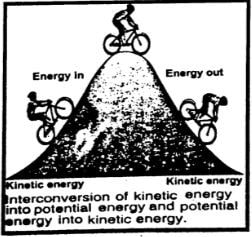2. Processes in nature are the results of energy changes. For example, some of the heat energy from the Sun is taken up by the water in the oceans. This increases the thermal energy.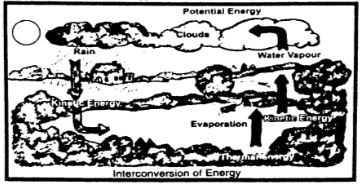Thermal energy causes water to evaporate from the surface to form water vapors. These vapors rise and form clouds. As they cool down, they form water drops and fall as rain. Potential energy changes to kinetic energy as the rain falls, this rainwater may reach a lake or a dam. As the rainwater flows down, its kinetic energy changes into thermal energy while parts of the kinetic energy of flowing water are used to wash away soil particles of rocks known as soil erosion.

Note: During the interconversion of energy from one form to other forms, the total energy at any time remains constant.

DO YOU KNOW?Pole vaulter:

A pole vaulter uses a flexible vaulting pole made of special material. It is capable to store all the vaulter's kinetic energy while bending in the form of potential energy. The vaulter runs as fast as possible to gain speed. The kinetic energy gained by the pole vaulter due to speed helps him/her to rise as the vaulter straightens. Thus, he attains height as the pole returns the potential energy stored by the vaulter in the pole.

List major sources of energy?

Difficulty: Easy

Major sources of energy:

1. Fossil fuels
2. Nuclear fuels
3. Energy from biomass
4. Energy from water
5. Energy from the sun
6. Solar house heating
7. Solar cells
8. Wind energy
9. Geothermal energy

Differentiate energy resources as renewable and non-renewable resources of energy with examples of each?

Difficulty: Easy

Renewable resources of energy:

Renewable sources of energy are those which can be reused. They do not get extinguished. They are environmentally friendly; they do not cause pollution.

Examples:

Solar energy, wind energy, and tidal energy.

Non-renewable resources of energy:

Non-renewable sources of energy are those which cannot be reused. They get extinguished. They cause pollution and are environmentally harmful.

List non-renewable sources of energy?

Difficulty: Easy
1. Fossil fuels
2. Nuclear fuels

Why are fossil fuels called a non-renewable form of energy?

Difficulty: Easy

Fossil fuels are nonrenewable resources because they took millions of years to attain their present form.

We use fossil fuels such as coal, oil, and gas to heat our houses, run industry, and transport. They are usually hydrocarbons (compounds of carbon and hydrogen). When they are burnt, they combine with oxygen from the air. The carbon becomes carbon dioxide; hydrogen becomes hydrogen oxide called water; while energy is released as heat. In the case of coal:

Carbon + Oxygen      $\rightarrow$  carbon dioxide + heat energy

Hydrocarbon + Oxygen    $\rightarrow$   carbon dioxide + water + heat energy

Describe the harmful waste products released by fossil fuels?

OR

List the environmental issues associated with fossil fuels:

Difficulty: Medium

Environmental issues associated with fossil fuels:

Fossil fuels release harmful waste products. These wastes include carbon monoxide and other harmful gases, which pollute the environment. This causes serious health problems such as headaches, tension, nausea, allergic reactions, and irritation of the eyes, nose, and throat. Long exposure to these harmful gases may cause asthma, lung cancer, heart diseases, and even damage to the brain, nerves, and other organs of our body.

Describe the processes by which energy is converted from one form to another concerning nuclear fuels?

OR

List the environmental issues associated with nuclear fuels?

Difficulty: Easy

Nuclear fuels:

In nuclear power plants, we get energy as a result of the fission reaction. During fission reaction, heavy atoms, such as Uranium atoms, split up into smaller parts releasing a large amount of energy.

Nuclear power plants give out a lot of nuclear radiation and a vast amount of heat. A part of this heat is used to run power plants while a lot of heat goes waste in the environment.

How energy is obtained from water?

Difficulty: Easy

Energy from water:

Energy from water power is very cheap. Dams are being constructed at suitable locations in different parts of the world. Dams serve many purposes. They help to control floods by storing water. The water stored in dams is used for irrigation and also to generate electrical energy without creating many environmental problems.

How energy is obtained from the sun?

Difficulty: Medium

Energy from the sun:

Solar energy is the energy coming from the Sun and is used directly and indirectly. Sunlight does not pollute the environment in any way. The sunrays are the ultimate source of life on Earth. We are dependent on the Sun for all our food and fuels. If we find a suitable method to use a fraction of the solar energy reaching the Earth, then it would be enough to fulfill our energy requirement.

Explain the functioning of the solar house heating system?

Difficulty: Medium

Solar house heating:

Complete solar house heating systems are successfully used in areas with a minimum amount of sunshine in winter. A heating system consists of:

1. A collector
2. A storage device
3. A distribution system

Working on solar heating system:

A Solar solar collector is made of glass panels over blank metal plates. The plates absorb the Sun's energy which heats a liquid flowing in the pipes at the back of the collector. The hot water can be used for cooking, washing, and heating the buildings.

Uses of solar energy:

Solar energy is used in solar cookers, solar distillation plants, solar powerplantst, etc.

Describe the process of electricity generated by solar cells?

Difficulty: Medium

Solar cells:

Solar energy can also be converted directly into electricity by solar cells. A solar cell also called a photocell is made from a silicon wafer.

When sunlight falls on a solar cell, it converts the light directly into electrical energy. Solar cells are used in calculators, watches, and toys. Large numbers of solar cells are wired together to form solar panels.

Solar panels can provide power to telephone booths, lighthouses, and scientific research centers. Solar panels are also used to power satellites.

Describe the process of electricity generated by wind energy?

Difficulty: Medium

Wind energy:

The wind has been used as a source of energy for centuries. It has powered sailing ships across the oceans. It has been used by windmills to grind grain and pump water.

More recently, wind power is used to turn wind turbines. When many wind machines are grouped on wind farms, they can generate enough power to operate a power plant.

In the United States, some wind farms generate more than 1300 MW of electricity a day.

Describe the process of electricity generated by geothermal energy?

Difficulty: Easy

Geothermal energy:

In some parts of the world, the Earth provides us with hot water from geysers and hot springs. There is a hot molten part, deep in the Earth called magma. Water reaching close to the magma changes to steam due to the high temperature of magma. This energy is called geothermal energy.

Geothermal wells can be built by drilling deep near hot rocks at places, where magma is not very deep. Water is then pushed down into the well. The rocks quickly heat the water and change it into steam. It expands and moves up to the surface. The steam can be piped directly into houses and offices for heating purposes or it can be used to generate electricity.

Describe the process of electricity generated by biomass?

Difficulty: Medium

Energy from biomass:

Biomass is plant or animal waste that can be burnt as fuel. Other forms of biomass are garbage, farm wastes, sugarcane, and other plants. These wastes are used to run power plants. Many industries that use forest products get half of their electricity by burning bark and other wood wastes. Biomass can serve as another energy source, but problems are there in its use.

When animal dung, dead plants, and dead animals decompose, they give off a mixture of methane and carbon dioxide. Electricity can be generated by burning methane.

State mass-energy equation $E = mc^{2}$

Difficulty: Easy

Mass-energy equation:

Einstein predicted the interconversion of matter and energy. According to him, a loss in the mass of a body provides a lot of energy. This happens in nuclear reactions. The relation between mass m and energy E is given by Einstein's mass-energy equation.

$E = mc^{2}$

Here c is the speed of light $(3\times10^{8} ms^{-1})$ The above equation shows that a tremendous amount of energy can be obtained from a small quantity of matter

Explain by drawing a flow diagram of electricity from fossil fuels.

Difficulty: Medium

Electricity from fossil fuels:

Most of the electricity is obtained using fossil fuels such as oil, gas, and coal. Fossil fuels are burnt in thermal power stations to produce electricity. Various energy conversion processes involved in producing electricity from coal are described in a block diagram.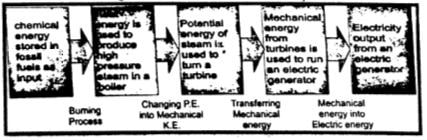List the environmental issues associated with energy. Highlight the solution to these issues?

Difficulty: Hard

Energy and environments:

Environmental problems such as pollution that consist of noise, air pollution, and water pollution may arise by using different sources of energy such as fossil fuels and nuclear energy.

Pollution:

Pollution is the change in the quality of the environment that can be harmful and unpleasant for living things.

Thermal Pollution:

A temperature rise in the environment that disturbs life is called thermal pollution. Thermal pollution upsets the balance of life and endangers the survival of many species.

Air pollutants:

Air pollutants are unwanted and harmful. Natural processes such as volcanic eruptions, forest fires, and dust storms add pollutants to the air. These pollutants, rarely build up to harmful levels. On the other hand, the burning of fuel and solid wastes in homes, automobiles, and factories releases a harmful amount of air pollutants.

The pollution produced by power plants and fission plants:

All power plants produce waste heat, but fission plants produce the most. The heat released into a lake, a river, or an ocean upsets the balance of life in them. Unlike other power plants, nuclear power plants do not produce carbon dioxide. But they do produce dangerous radioactive wastes.

Controlling pollution issues:

In many countries, governments have passed laws to control air pollution. Some of these laws limit the amount of pollution that, power plants, factories, and automobiles are allowed to give off. To meet these conditions for automobiles, new cars have catalytic converters. These devices convert some polluting gases. The use of lead-free petrol has greatly reduced the amount of lead in the air. Engineers are working to improve new kinds of car engines that use electricity or energy sources other than diesel and petrol.

Many individual communities have laws that protect their areas from pollution. Individuals can help to control air pollution simply by reducing the use of cars and other machines that burn fuel. Sharing rides and using public transportation are ways to reduce the number of automobiles in use.

Explain by drawing energy flow diagrams through a steady-state system such as a filament lamp a power station, or a vehicle traveling at a constant speed on a level road?

Difficulty: Easy

Flow diagram of an energy converter:

In an energy converter, a part of the energy taken (used up) by the system is converted into useful work. The remaining part of the energy is dissipated as heat energy and sound energy (noise) into the environment.

The energy flow diagrams given below show the energy taken up by an energy converter to transform it into other forms of energy.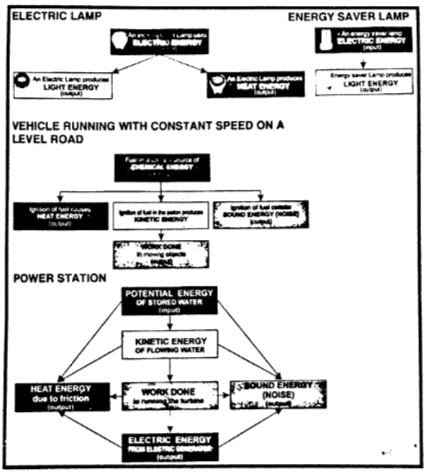What is meant by the efficiency of a system?

OR

How can you find the efficiency of a system?

Difficulty: Medium

Efficiency:

The ratio of the useful work done by a device or machine to the total energy taken up by it is called its efficiency.

OR

The efficiency of a system is the ratio of the required form of energy obtained from a system as output to the total energy given to it as input.

Efficiency= $\frac{(required form of output)}{(total input energy)}$..... (i)

% Efficiency= $\frac{ (required form of output)}{(total input energy)}×100$ ......(ii)

Ideal system/Ideal machine:

An ideal system is that which gives an output equal to the total energy used by it. In other words, its efficiency is 100 %.

People have tried to design a working system that would be 100 % efficient. But practically such a system does not exist.

 Efficiencies of some typical devices/machines Energy Input Device or machines Useful Work done % Efficiency 100 J Electric lamp 5 J 5 % 100 J Patrol Engine 25 J 25 % 100 J Electric Motor 80 J 80 % 100 J Electric Fan 55 J 55 % 100 J Solar Cell 3 J 3 %

What is meant by the term power? Define watt.

Difficulty: Medium

Power is defined as the ratio of doing work.

Mathematically,

Power                 P= $\frac{Work \: done}{Time \: taken}$

Or                      P= $\frac{W}{t}$………… (i)

Since work is a scalar quantity, therefore, power is also a scalar quantity.

Note:     Bigger units of power are kilowatt (kW), megawatt (MW), etc.

1 kW=1000W=10^{3}

1MW=1000 000=10^{6}

1 horsepower= 1hp = 746 W